### Home > CC3 > Chapter 7 > Lesson 7.3.2 > Problem7-106

7-106.
1. Simplify and solve the following equations. Homework Help ✎

1.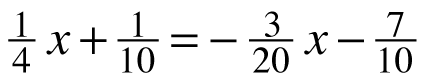2. 3x + 4.5 = 4.5x − 18

3.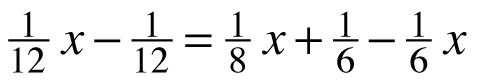4. 6.25x + 7.5 − 2.5x = 3.75x − 8.75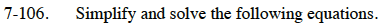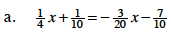Find a common denominator to get rid of the fractions.
Use 20.
5x + 2 = −3x − 14

Combine like terms and solve for x.

x = −2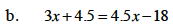Combine like terms and solve for x.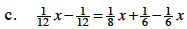Refer to (a).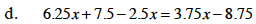Refer to (b).